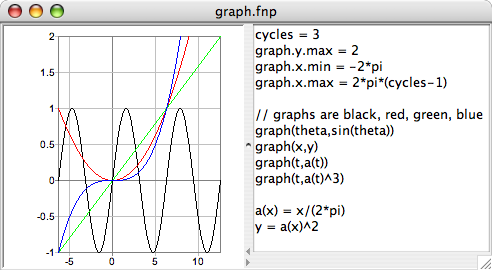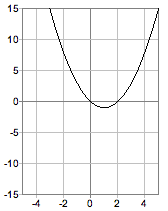fnPad (pronounced "fun-pad") is a text editor based, graphing, programmable calculator. Arithmetic expressions are entered in a text window, and when calculated, by selecting the "Calculate" menu item, the results are inserted into the text following the expressions.

CONTENTS

The "jar" file [do whatever you've got to do with your browser to download the linked file] of version 0.32 (2010 Jan 9) can be downloaded. You need to have version 1.5 or higher of the Java™ Runtime Environment (available for Windows and Mac OS X). On some systems (e.g., Mac OS X), you can open fnPad.jar as you do other files, e.g., by double-clicking it. It can be run from a shell by the command "java -jar fnPad.jar".

Mac users who are disoriented by having menus in the window instead of the menu bar, and by a funky application name can use the rather hideous shell command

```\$ java -Dapple.laf.useScreenMenuBar="true" \
```

#### examples

The syntax will be familiar to some, and is pretty simple anyhow. A little example follows.

```// A comment starts with a double slash
// and ends with the line.
3+8 ≈ 11 // Add two numbers.
// fnPad inserts the wavy equals
// and the result which follows it
// when the "Calculate" menu item is selected.

// Do multiple operations in an expression.
2+3*7 ≈ 23

// Parentheses override the normal precedence
// or associativity of operators.
(2+3)*7 ≈ 35

// Division of integers results in an integer.
5/2 ≈ 2

// Division of floating point numbers
// or mixed floating point and integers
// results in a floating point number.
5./2 ≈ 2.5

// An expression ends with the line or at a semicolon.
16./5. ≈ 3.2; 2^5 ≈ 32.0

// Note that all of these calculations are done at once.
```

More interesting things can be done by using definitions.

```// A definition can be an identifier = an expression.
x = 3

// Things that are defined can be used in an expression.
y = x+1

// Definitions can have parameters
// and occur in the text after being used.
b = length(x,y)

// Note that this "b" has a different value
// than "b" in the "length(a,b)" definition.
b ≈ 5.0
length(b,12) ≈ 13.0

// There are some included functions and predefined constants.
atan2(y,x)*180/pi ≈ 53.1301

// Here's a function definition, length of the hypotenuse,
length(a,b) = sqrt(a^2+b^2)

// and another, base 10 logarithm.
log(x) = ln(x)/ln(10)

log(100) ≈ 2.0; log(0.1) ≈ -1.0
```

Definitions can be recursive, in which case a conditional expression is needed.

```// Here are a couple of conditional expressions.
// The first one means: if x is less than 0,
// then the result is -x, otherwise the result is x.
x < 0 ? -x : x ≈ 1  // absolute value
x >= 0 ? x : 0 ≈ 0  // clip below zero

x = -1

// a recursive definition of the greatest common divisor
gcd(a,b) = ( a%b == 0 ) ? b : gcd(b,a%b)
//    This ↑__________↑ parenthesis is for clarity.

gcd(648,156) ≈ 12
```

Any of the example text can be copied and pasted into a fnPad window.

#### graphing

An example of graphing is shown in the image of a fnPad window below. See that graph(x,f(x)) is used to produce a graph of some function, where the first argument is some symbol (variable name) and the second argument is some expression that (usually) is a function of that variable.

The extent of the coordinates may be specified, as in the example, with definitions of graph.x.min (for the minimum of the horizontal axis) and so on. If undefined, the extent of each axis is -1 to 1.

The color of each graph depends on the order of the graph() applications in the text. The light gray lines are the coordinate axes.To see the graph, the left-hand pane of the window must be opened, either by dragging the divider, or clicking on the "move divider right" control (if there is one in the interface).

As can be seen, graph(x,f(x)) is different than other functions in two ways: it has the side effect of drawing in the graph panel, and it has no result. Another, less obvious way in which graph() is different is that its arguments are not directly evaluated when it is applied, but its second argument, f(x), is evaluated over a range of values for its first argument, x.

Below is an example that finds the roots of a quadratic equation and graphs the parabola. Try changing the coefficients and seeing how the graph changes. [If you change the coefficients by too much you might also need to change the bounds of the graph to see the parabola.]```// To see the graph,
// open the left panel.

// Solve and graph
// Define the coefficients.
a = 1; b = -2; c = 0

// Find the discriminant and roots.
d ≈ 4.0; root1 ≈ 0.0; root2 ≈ 2.0

graph(x,a*x^2+b*x+c)

// Define the graph's bounds.
graph.x.min =  -5; graph.x.max =  5
graph.y.min = -15; graph.y.max = 15

root1 = (-b-sqrt(d))/(2*a) // root with -
root2 = (-b+sqrt(d))/(2*a) // root with +
d = b^2-4*a*c // discriminant
```

There's another page with a more extensive example that applies the quadratic formula.

#### operators

The following are all the operators, lowest to highest precedence. Association is left, except for "^", e.g., a/b/c = (a/b)/c, a^b^c = a^(b^c).

• definition
• =
• conditional
• ? :
• boolean
• && (and) || (or)
• ! (not)
• comparison
• < > != <= == >=
• arithmetic
• + -
• * / % (modulus)
• ^ (exponentiation)
• - (unary minus)
• bits
• & (and) | (or) \$ (exclusive or)
• << (left shift) >> (right shift)
• ~ (complement)

#### functions

The following functions are included. The trigonometric functions use radians.

• sin(theta) - sine of an angle
• cos(theta) - cosine of an angle
• tan(theta) - tangent of an angle
• asin(r) - arcsine of a number
• acos(r) - arccosine of a number
• atan(r) - arctangent of a number
• atan2(y,x) - arctangent of y/x
• ln(r) - natural logarithm of a number
• sqrt(r) - square root of a number
• abs(r) - absolute value of a number
• rnd(r) - a number rounded to the nearest integer
• hex(i) - a hexadecimal string of an integer
• oct(i) - an octal string of an integer
• fix(r,n) - a fixed point decimal string of a number with specified precision
• eng(r,n) - a floating point decimal string of a number with specified precision

Other functions, written in Java, can be added by deriving from the Function class. See cos.java for an example.

#### predefined constants

The following "constants" are predefined.

• pi
• e
• true
• false

There are some missing basic things that need to be added.

• printing - use another text editor to print
• setting and saving preferences
• catching a Quit from the Mac Jar Runner

Here are the next planned major features.

• arrays